# Projectile motion problems

1. Here are Multiple Choice Questions(More than one correct) for Projectile motion problems with detailed solution.
2. Recommended way is to solve them on your own and then check solutions for correctness

Question 1
A ball is projected upward at a certain angle with the horizontal .which of the following statement is/are correct. At highest point
a. velocity of the projectile is not zero
b. acceleration of the projectile is zero
c. velocity of the projectile is along the horizontal direction
d. Acceleration of the projectile is vertically upwards

Question 2
Which of the following remains constant during the motion of the projectile fired from a planet,
a. KE
b. Momentum
c. Vertical component of the velocity
d. Horizontal component of the velocity

Question 3
Which one is wrong for a body having uniform circular motion?
a. Speed of the body is constant
b. Acceleration is directed towards the centre
c. Velocity and Acceleration vector are having an angle 45
d. none of the above

Question 4.
The range of the projectile depends upon
a. Angle of the projection
b. Acceleration due to gravity
c. Mass of the projectile
d. magnitude of the velocity of projection

Question 5.
Two bullets A and B are fired horizontally with speed v and 2v respectively. Which of the following is true a. Both will reach the ground in same time
b. Bullet with speed 2v will cover more horizontal distance on the ground
c. B will reach the ground in less time than A
d. A will reach the ground in less time than B

Question 6.
A body is projected horizontally from a point above the ground.The motion of body is defined as
$x=2t$
$y=2t^2$
where x and y are horizontal and vertical displacement respectively at time t.Which one of the following is true
a. The trajectory of the body is a parabola
b. The trajectory of the body is a straight line
c. the velocity vector at point t is 2i+4tj
d. the acceleration vector at time t is 4j

Question 7.
The initial velocity of the particle is u=4i+3j m/s. It is moving with uniform acceleration a=.4i+.3j m/s2.which of the following is true
a. the magnitude of the velocity after 10 sec is 10m/s
b. The velocity vector at time t is given by (4+.4t)i +(3+.3t)j
c. the displacement at time t is (4t+.22)i +(3t+.152)j
d . None of the above

Question 8.
Two projectile A and B are having trajectory equation
$y=a_1x-b_1x^2$ and $y=a_2x-b_2x^2$
If the range is same for both the projectile A and B then which of the following option is true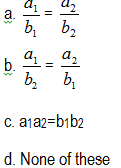Question 9.
When the projectile is at the highest point of its trajectory, the direction of its velocity and acceleration are
a. parallel to each other
b. anti parallel to each other
c. Inclined to each other at 45
d. Perpendicular to each other

Question 13
The horizontal and vertical displacement of the projectile at time t are
$x=36t$
$y=48t-4.9t^2$
where x and y are in meters and t in second. Initial velocity of the projectile in m/s
a. 15
c. 30
b. 45
d. 60

Question 14.
It is possible to project an body with a given speed in two possible ways so that it has a same horizontal range. The product of time taken by it in tow possible ways is
a. $\frac {R}{g}$
b. $\frac {2R}{g}$
c. $\frac {3R}{g}$
d. $\frac {4R}{g}$

Question 15.
A projectile has a range R and time of flight T. If the range is tripled by the increasing the speed of the projection, without changing the angle of projection then the time of the flight will become
a $\frac {T}{\sqrt {3}}$
b $T \sqrt {3}$
c.$\frac {T}{3}$
d. $3T$

Question 16.
A large number of stones are fired in all the direction with same speed V.The maximum area of the ground on which this stone will spread is
a. $\frac {\pi V^4}{g^2}$
b. $\frac {\pi ^2V^4}{g^2}$
c. $\frac {\pi V^4}{g}$
d. $\frac {\pi V}{g^2}$

Question 17.
A body is projected horizontally from the top of the building 39.2 m high. How long will it take to hit the ground
a. 2 sec
b. 4 sec
c. 1 sec
d. $2 \sqrt {2}$ sec

Question 18
A boy throws a ball with such an initial velocity and at such an angle of elevation, so that the range is r and maximum height to which ball rises is h. find the maximum range that can be obtained with same initial velocity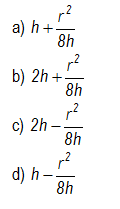Question 19.
An airplane is moving with a horizontal velocity v at a height h above a level plane.If a projectile is fired from an gun at an instant when the plane is vertically above the gun, what is the minimum initial velocity of the projectile in order to hit the plane.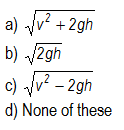Question 20.
An aero plane is moving in the sky and a bag is dropped from t he plane. Let a and v denote the acceleration and velocity of the aero plane. Consider two cases
1. a is constant and non zero
2. a is zero and plane is moving with uniform velocity
Match the following:-
 (P) The trajectory of bag in case A w.r.t. ground is (L) Parabola (Q) The trajectory of bag in case B w.r.t. plane is (M) straight line (R) The trajectory of bag in case B w.r.t. ground is (N) no appropriate match (S) The trajectory of bag in case A w.r.t. plane is (O) Circle

Question 21.
An object under goes an projectile motion starting from origin with initial velocity
Under acceleration due to gravity
Hm  -> Maximum height reached by object
R -> Range of the object
Match the column
 Column A Column B A) tan of angle between velocity and x axis at any point of time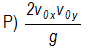B) tan of projection angle θ0 for a projectile at origin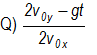C) Range of the projectile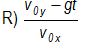D) tan of the angle of position vector at any time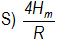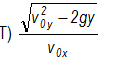## Passage type Questions

(A) A plank of length L is moving in the direction of x-axis in the X-Y plane. A point A is located on the left hand side of the plank. Position of point A at t=0 is (L,0). The plank is moving in the right direction with velocity v
Question 22.
A person standing at point A at t=0 throws a stone with velocity v and at an angle 600 with X axis. Find the co-ordinates of the maximum height reach by the stone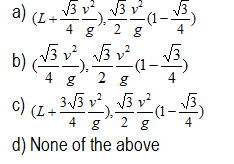Question 23.
Find the velocity vector at the maximum height if i and j  are the unit vectors along X and Y axis respectively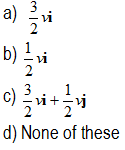Question 24.
Find the co-ordinates of the stone where its hit the X-axis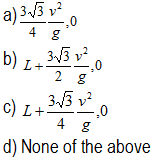Question 25.
Find the velocity vector of the stone when it hits X-axis.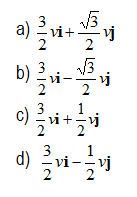Question 26.
Find the co-ordinates of the point A on the plank when the stone hit the X-axis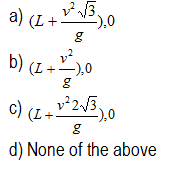Question 27.
Find the maximum value of velocity v so as to land the stone on the plank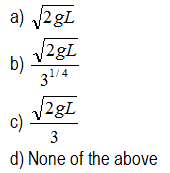(B)An object is fired up on the inclined place of angle $\alpha$ . The object is fired with velocity $v_0$ and angle of elevation $\theta$ with the horizontal
Question 28.
Find the range on the inclined plane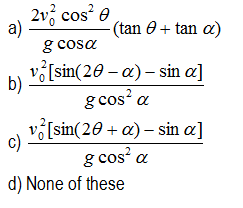Question 29.
Find the relation between $\theta$ and $\alpha$ for which object will have maximum range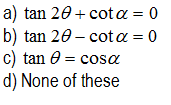Question 30.
A stone is thrown from ground level over horizontal ground. It just clears three walls, the successive distances between them being r and 2r. The inner wall is 15/7 times as high as the outer walls which are equal in height. The total horizontal range is nr, where n is an integer. Find n
a. 2
b. 3
c. 4
d. 5